# Analog LTC2311-16 3v01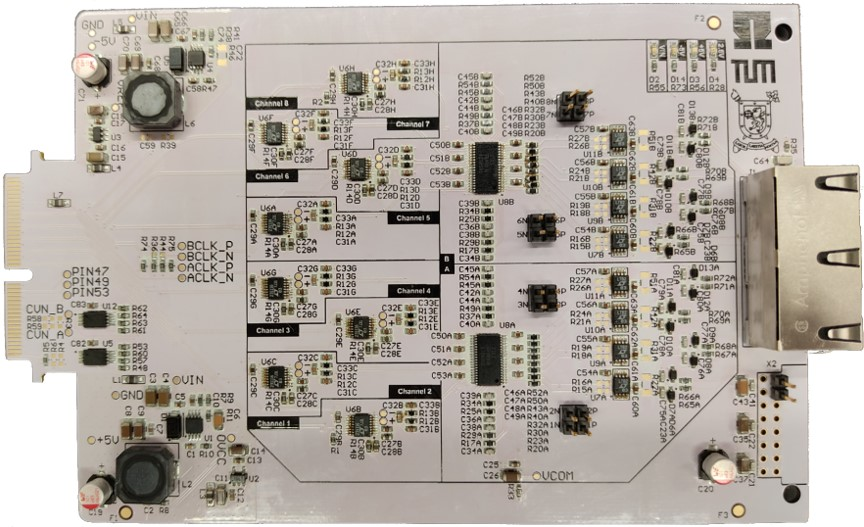## Diagrams

Block diagram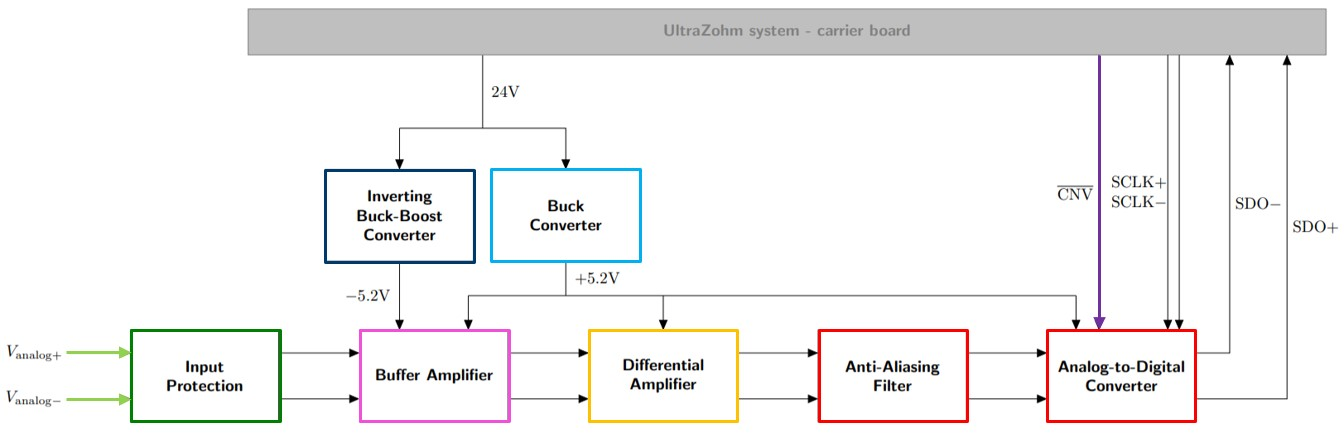Schematic excerpt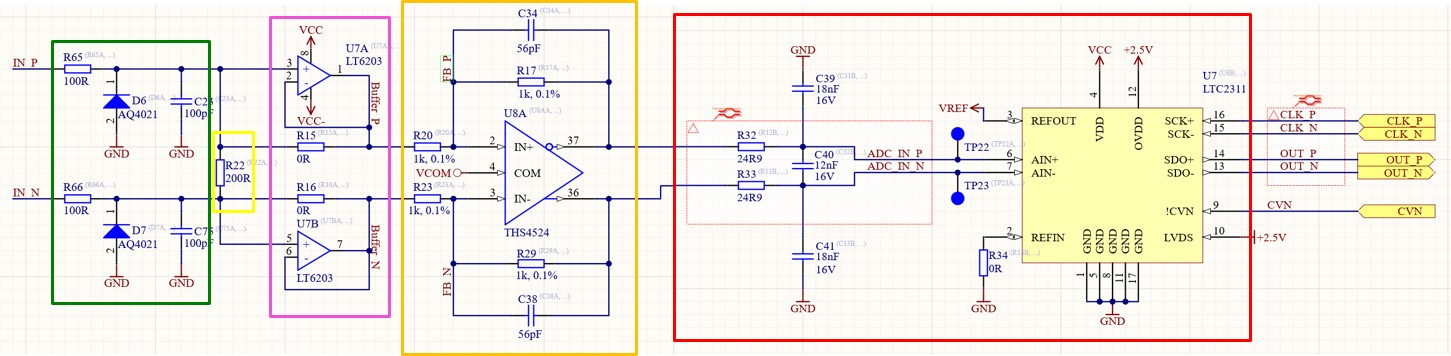PCB colored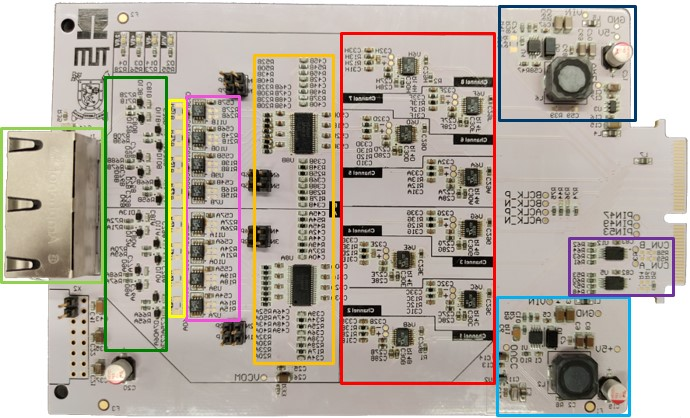From left to right

Notice that the picture of the PCB is flipped to match the orientation of the block diagram and schematic.

Color

Functionality

light green

dark green

Input protection (ESD diodes)

yellow

Measurement shunt resistor (optional, not populated)

pink

orange

Differential OpAmp to scale and filter signals

red

dark blue

Inverting buck-boost converter for -5V

light blue

Buck converter for +5V

purple

Buffer for CNV signal coming from the FPGA

## Filters

1. The crossover frequency $$f_\mathrm{aliasing,-3dB}$$ of the anti-aliasing filter (red) is formed by the capacitors $$C_\mathrm{Diff}$$ and $$C_\mathrm{CM}$$. They create a first order low-pass filter with the series resistors $${R_0}$$ with

$f_\mathrm{aliasing,-3dB}=\frac{1}{2 \pi R_0 (2 C_\mathrm{Diff} + C_\mathrm{CM} ) } = \frac{1}{2\pi\ 24.9\,\Omega \cdot (2\cdot16\,\mathrm{nF} + 12\,\mathrm{nF}) } = 145\, \mathrm{kHz} \,\,.$
1. Additionally, $$C_\mathrm{f}$$ and $$R_\mathrm{f}$$ form an active first-order low-pass filter, with

$f_\mathrm{DiffOp,-3dB} = \frac{1}{2\pi R_f C_f} \approx \, 3 \mathrm{MHz}$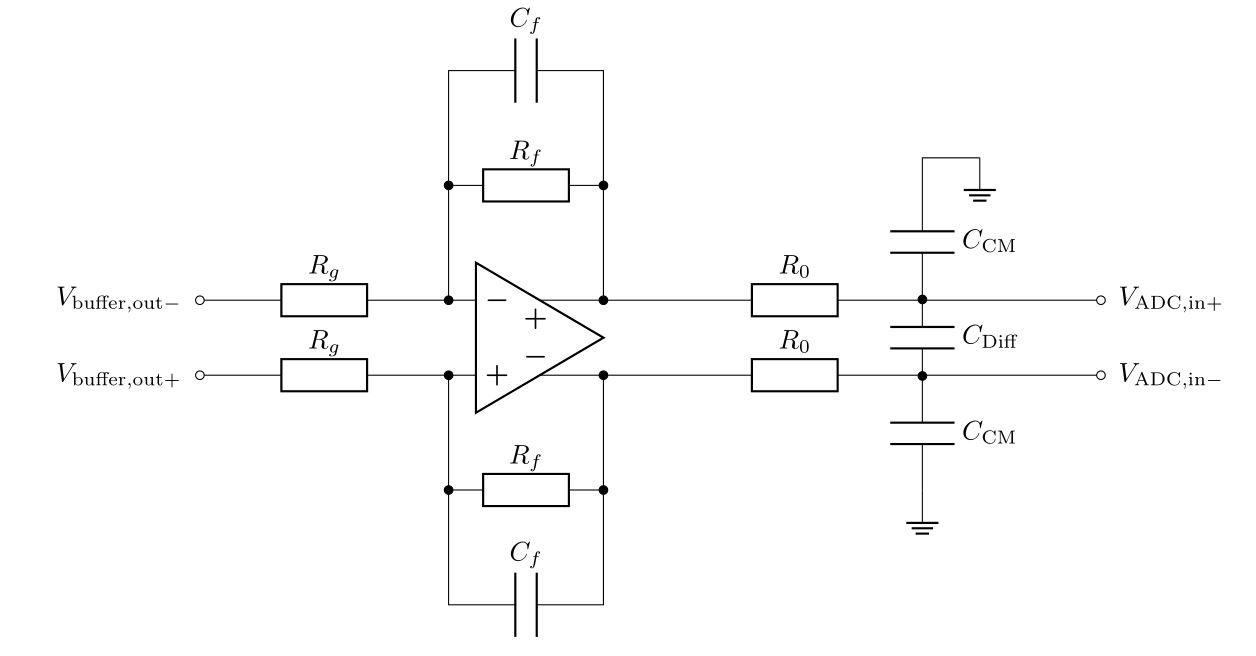The resulting transfer function of the signal conditioning stage, i.e., from the RJ45 input connectors to the $$V_\mathrm{ADC,in}$$ (including the buffer and filter stages up to the point where the signal enters the ADC), is measured with the Bode100. The resulting bode plot is depicted below.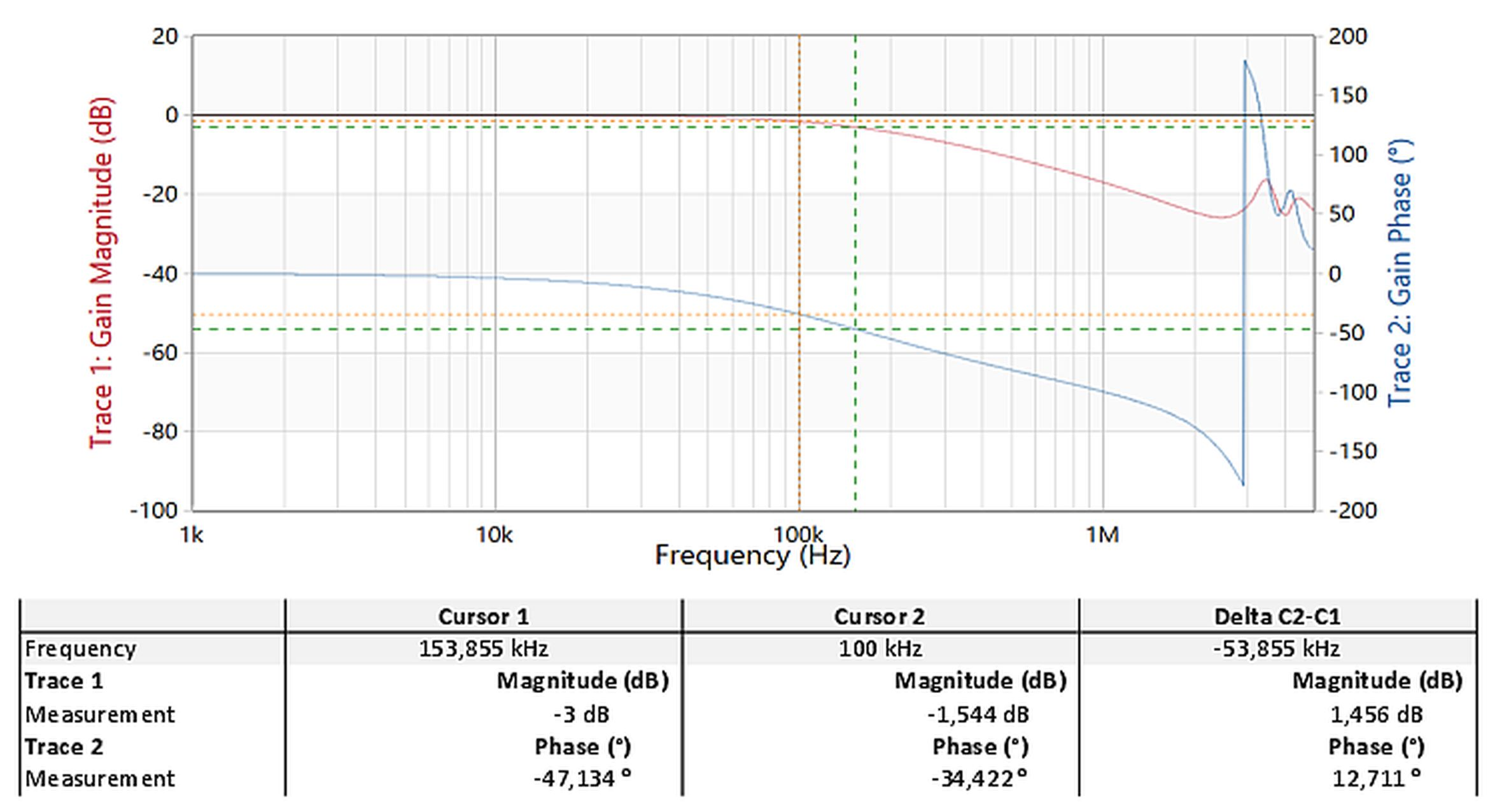## Measurement accuracy

The signal conditioning stage of the adapter card is validated with a 6 1/2 digit multimeter yielding the following results. This suggests that a measurement error below 2mV can be expected over the full input range.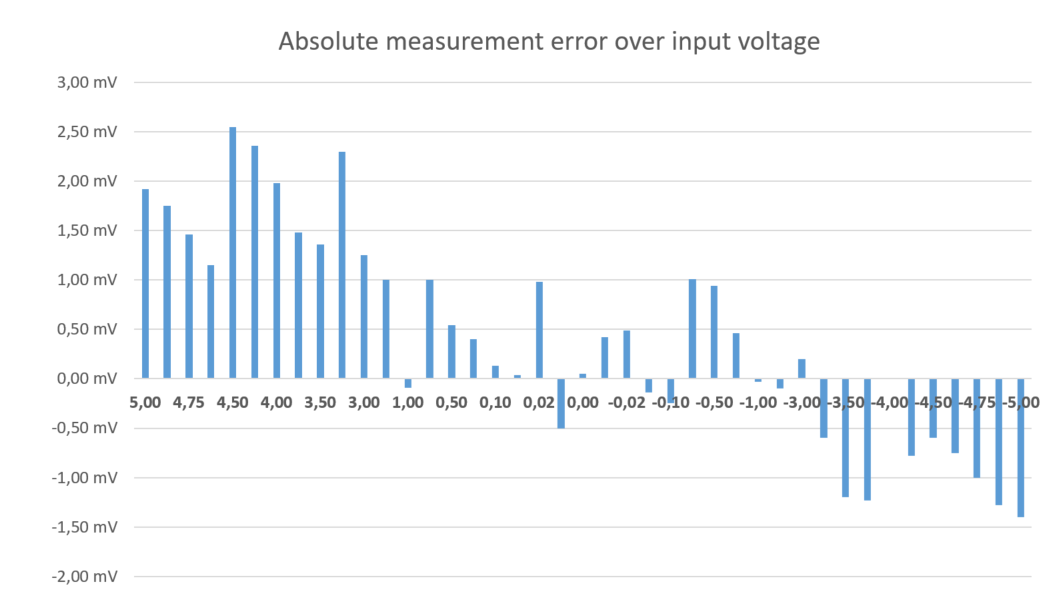For all types of measurements, described in Measurement modes, it is recommended to adjust the gains of the differential operational amplifier (orange) to utilize the full input range of the ADC, i.e., $${\pm 5}$$ V. Initially, the gain is set to

$g=\frac{R_f}{R_g} = \frac{1\,\mathrm{k\Omega}}{1\,\mathrm{k\Omega}} = 1 \,\,.$

Note

Use a high precision resistor to set the gains, i.e., 0.1% or better.

The pre-assembled values are

 $${R_f}$$ $${1\,\mathrm{k\Omega}}$$ $${R_g}$$ $${1\,\mathrm{k\Omega}}$$ $${C_f}$$ $${56\,\mathrm{pF}}$$ $${R_0}$$ $${24.9\,\mathrm{\Omega}}$$ $${C_\mathrm{CM}}$$ $${12\,\mathrm{nF}}$$ $${C_\mathrm{Diff}}$$ $${16\,\mathrm{nF}}$$

Note

You can also use the LTSpice simulation to find the correct gains and filter values for your setup. The simulation files are attached at the end of this document.

## Compatibility

• Slots A1 to A3 can be used without limitations